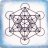# Historical Earnings Trend Indicator for ThinkorSwim

B

#### beh0211

##### New member
Inspired by this post, I am creating a similar indicator but it show EPS growth comparing to the same quarter last year.

It may not looks good but it is workingCode:
``````#Only works in weekly chart

declare lower;

def EPS = if !isNaN(GetActualEarnings()) then GetActualEarnings() else EPS;

def EPSGrowthYoY = if !isNaN(GetActualEarnings()) then round(100*(EPS - EPS)/AbsValue(EPS), numberOfDigits = 0) else EPSGrowthYoY;

plot pEPSGrowthYoY = EPSGrowthYoY;
pEPSGrowthYoY.SetDefaultColor(color.blue);

plot pZero = 0;
pZero.setDefaultColor(Color.dARK_GRAY);
pzero.setLineWeight(3);

AddLabel(yes, if EPSGrowthYoY!=0 then "EPSGrowthYoY% = " + EPSGrowthYoY+"%" else "EPSGrowthYoY% = " + EPSGrowthYoY + "%", color = Color.Blue);

# end code``````

Last edited:
•Bamilus
N

#### Nick

##### Active member
2019 Donor
VIP
@J007RMC @BenTen

Thanks for your sharing. I have tried to use the two scripts shared and modify it to only show the Gain and the difference of earnings by each quarter in % in each bubble. I couldn't find the reference to get the previous earnings for comparison.
May I request some assistance here.
The % change in earnings per quarter is calculated by (current earnings - previous earnings ) divided by previous earnings
If current quarter earning is higher that prev quarter = Green bubble
if current quarter is lower than prev quarter = Red bubble

Thank you

Code:
``````def price = close;
def estimate = GetEstimatedEarnings();
def actual = GetActualearnings();
def get_price = if estimate and actual then price else get_price;
def gain = (actual – estimate);
AddChartBubble(estimate,close, “Est: ” + estimate + “\nAct: ” + actual + (if gain <= 0 then "\nLoss: " else “\nGain: ”) + gain, if actual <= estimate then CreateColor(247, 25, 91) else CreateColor(25, 202, 247));``````•Bamilus
R

#### ReyHak

##### New member
Hi,
I like to add a few columns for the past few quarters/years of actual EPS, how can I do this please?Staff
VIP

R

#### ReyHak

##### New member
Thanks @BenTen I did look through this earlier but these are all chart indicators, I like to have this as a column on my watch list, can you help please?Using External Historical Data in ThinkorSwim? Questions 7